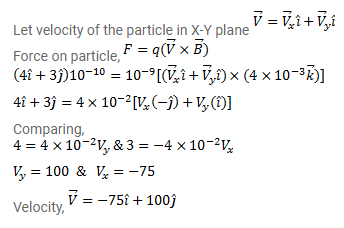# A magnetic field of

Question:

A magnetic field of $\left(4.0 \times 10^{-3} \vec{k}\right)$ T exerts a force of $(4.0 \vec{\imath}+3.0 \vec{\jmath}) \times 10^{-10} \mathrm{~N}$ on a particle having a charge of $1.0 \times 10^{-9} \mathrm{C}$ and going in the $X-Y$ plane. Find the velocity of the particle.

Solution: